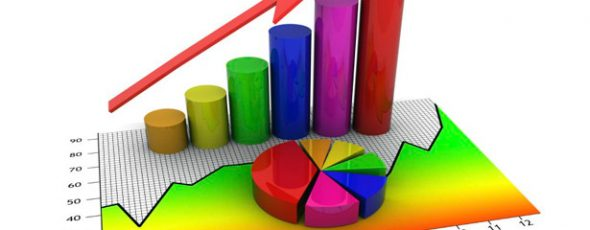# Statistical Tools and when to use them?

JoshuaSelecting the appropriate statistical tools (eg. Correlation, Regression) for the data you have collected can be a complicated task. However, once you understand the nature of your data, the way you wish to present it and the type of results that you require, selection of the tests that you need for the analysis is fairly simple.

Here are some of the most common tests that you will see carried out during research and when to use them.

## 1.Understanding the Relationship Between variables

 Tool When to use it Example Cross-tabulations To find the quantitative relationship between different variables. Analysis of the gender-based differences between the various religions in different generations of immigrants. Chi-Square test To find out if there is a significant relationship between categorical variables. It can be used to find the association between the variables and to examine hypotheses. Examination of whether there is a significant gender-based difference in preference for one of two colours. Correlation analysis To determine if there is a relationship between two variables and measure the strength of the association. Determination of a relationship between the environmental temperature and increase in ice cream sales. Pearson’s Correlation To determine if there is a linear relationship between two variables and measure the strength of this association. Examination of the relationship between height and hypertension in a patient. Spearman Correlation To determine if there is a relationship between two ordinal variables and measure the strength of the relationship. Spearman’s correlation does not rely on the data being normally distributed. Examination of the relationship between the level of highest education received & initial salary provided in an organization.

## 2.Finding the Difference Between the Means

 Tool When to use it Example Independent t-tests To determine if there is a significant difference between the means of two independent groups. Determining if there is a significant difference between the heights of the two genders. Paired t-tests To determine if there is a significant difference between the means of two related groups, where the data is normally distributed and continuous. Pre- and post-test diagnostic scores for two subjects ANOVA To determine if there is any difference between the sample means of groups. Examination of weight loss effects of three types of diet pills. Mann-Whitney U test To identify significant differences between the means of ordinal variables. It is a non-parametric test. Comparison of reaction times between two groups of patients, each having taken a specific medicine.

## 3. Determining Whether a Change in one Variable can Predict a Change in Another Variable

 Tool When to use it Example Linear Regression To determine if the change in one variable can predict a corresponding change in another variable. Determination of whether an increase in age affected consumption of junk food. Multiple Regression To determine if a change in two or more variables can bring about changes in the outcome. Determination of factors affecting the health of a plant.

## 4.Factor Analysis

Factor analysis is a commonly used statistical tool to determine whether a given variable can be discussed using selected factors. Two types of factor analysis are generally discussed, including exploratory factor analysis and confirmatory factor analysis.

Exploratory factor analysis is generally used as a preliminary measure to test the underlying theory. In this case, the exact number of factors is not known.

Confirmatory factor analysis determines whether the variables that were measured in the given study can be used to represent the given constructs. This test provides an insight as to which factors affected the final outcome and the weightage that each of the variables has on the outcome.

This is just a basic introduction to some of the tests you may be considering using. There are a number of other statistical tools that can be adopted, including post-hoc tests that are carried out on completion of these tests.

Are you confused about the statistical tools that you want to use for your analysis? Are you unsure if the tests that you have used are correct? For more support and guidance, please feel free to contact us at +91 9731988227. If you are curious about the statistical tools we use, click here.

Rehoboth Academic Services is a premium institute supporting PhD & Master’s Thesis. We offer editing, proofreading, writing, statistical analysis, formatting and plagiarism checking services. We have helped more than 1000+ research scholars in most of the subjects and universities across the globe in the last seven years. We also conduct Copyediting & Proofreading, Art of thesis writing, Research Paper writing, Statistical Analysis with SPSS Foundation & Advanced workshops. If you need our assistance please contact us

9731988227, 9741871657